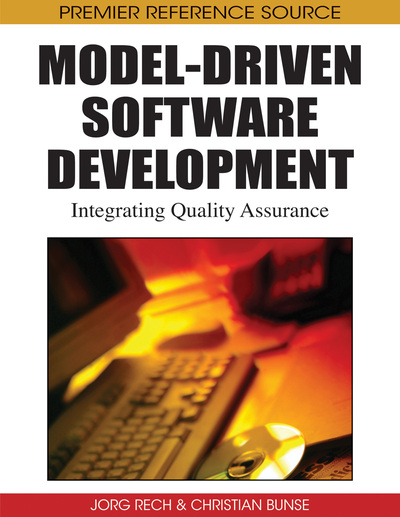# A Pattern Approach to Increasing the Maturity Level of Class Models

Michael Wahler (IBM Zurich Research Laboratory, Switzerland)
DOI: 10.4018/978-1-60566-006-6.ch009

## Abstract

Class models are typically specified at a high level of abstraction and subsequently refined with textual constraints to obtain higher maturity levels. This task requires significant expertise and effort because constraints must be elicited and formalized. In this chapter, we identify typical refinement problems for class models that threaten model quality and show how a list of refinement tasks can be automatically compiled from a given model. We present constraint patterns that help to carry out these tasks semiautomatically and introduce a tool prototype for our approach.
Chapter Preview
Top

## 1. Introduction

Models have been used in software engineering for illustration and documentation purposes for a long time. In the past few years, model-driven development approaches such as Model-Driven Engineering (MDE) have become popular, and with these approaches, models have enormously gained importance. Still used for illustration, models now also serve as semantic foundation for the development of whole systems in certain domains: they are abstract representations of various aspects of a system and drive the development process. This new role requires a certain degree of formality for the syntax and the semantics of models: Whereas it was sufficient for the syntax and the semantics to be intuitively understandable in the age of “models-as-illustrations”, they need to be formally specified in the MDE age because they are the basis of the generated code.

In MDE, the abstract syntax of a modeling language is defined by a meta-model, which is usually a class model that graphically specifies the elements of a modeling language, such as classes and their properties, and the dependencies between these elements. The dependencies between classes are especially important in this refinement: Since each model that complies with its meta-model must be a valid abstraction of the system, the dependencies between elements in the model must be precisely captured in the meta-model. Whereas these dependencies can only be coarsely constrained within the graphical model itself, textual constraint languages such as the Object Constraint Language (OCL) (Object Management Group (OMG), 2003) are used to express details about the dependencies.

Adding textual constraints to a class model rules out invalid instances, which increases the maturity level of the class model (Kleppe and Warmer, 2003). For visualizing this idea, we use a function I that maps a set of concepts to the set of all possible objects for these concepts. In particular, I(M) denotes the set of all objects in all possible instances of a model M and I(R) denotes the set of all possible objects in a real system. Figure 1 a) visualizes a model M with a low maturity level: A large part of I(M) is not inside I(R), i.e., I(M) contains many elements that are not representations of the real system. By adding constraints to M, a model M’ can be developed with a higher maturity level. Figure 1 b) shows that there are less elements in the set I(M’) − I(R), which means that significantly less invalid instances can be derived from M’ than from M. Thus, M’ has a higher maturity level than M.

In this chapter, we focus on increasing the maturity level of models as one important quality aspect. Thus, the aim of this chapter is to identify a number of causes for low maturity levels of models in early stages of development, show how these causes can be found in a given model, and offer solutions to refine the model and increase its maturity level. We focus on reducing the size of I(M)−I(R) and provide general guidelines for creating class models of a high maturity level and thus, a high quality.

In order to refine a given class model, it must be thoroughly analyzed by the model developer. However, not all causes for low maturity may be detected manually, which can cause serious problems in the MDE process because the generated code may cause runtime exceptions. To simplify and partly automate model analysis, we have identified recurring problems in class models that require refinement by textual constraints. The first objective of this chapter is to present these problems, show examples of how they threaten the maturity of class models, and introduce tool support for their automatic detection.

## Complete Chapter List

Search this Book:
Reset